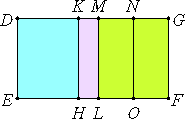# Proposition 63

The square on the major straight line applied to a rational straight line produces as breadth the fourth binomial.

Let AB be a major straight line divided at C, so that AC is greater than CB, let DE be a rational straight line, and to DE let there be applied the parallelogram DF equal to the square on AB and producing DG as its breadth.

I say that DG is a fourth binomial straight line.

Make the same construction as shown before.X.39

Since AB is a major straight line divided at C, therefore AC and CB are straight lines incommensurable in square which make the sum of the squares on them rational, but the rectangle contained by them medial.

X.20

Since the sum of the squares on AC and CB is rational, therefore DL is rational. Therefore DM is also rational and commensurable in length with DE.

X.22

Again, since twice the rectangle AC by CB, that is, MF, is medial, and it is applied to the rational straight line ML, therefore MG is also rational and incommensurable in length with DE.

Therefore DM is also incommensurable in length with MG. Therefore DM and MG are rational straight lines commensurable in square only. Therefore DG is binomial.

It is to be proved that it is a fourth binomial straight line.

In manner similar to the foregoing we can prove that DM is greater than MG, and that the rectangle DK by KM equals the square on MN.

Since the square on AC is incommensurable with the square on CB, therefore DH is also incommensurable with KL, so that DK is also incommensurable with KM.

X.18

But, if there are two unequal straight lines, and to the greater there is applied a parallelogram equal to the fourth part of the square on the less and deficient by a square figure, and if it divides it into incommensurable parts, then the square on the greater is greater than the square on the less by the square on a straight line incommensurable in length with the greater, therefore the square on DM is greater than the square on MG by the square on a straight line incommensurable with DM.

And DM and MG are rational straight lines commensurable in square only, and DM is commensurable with the rational straight line DE set out.

X.Def.II.4

Therefore DG is a fourth binomial straight line.

Therefore, the square on the major straight line applied to a rational straight line produces as breadth the fourth binomial.

Q.E.D.

## Guide

This proposition is used in X.72.